## Vector control of Induction motor

##### Introduction

In this experiment, a mathematical model of an induction motor will be simulated based on the parameters estimated in the previous experiment. For this, unlike the previous experiment where the model was in abc domain, the motor will be modeled in dq frame. With proper alignment of the dq frame, the motor model becomes simpler compared to motor modelled in abc frame. The major advantage of this approach, other than the reduction in simulation run-time, is the ease of PI controller design to control motor speed, current or position. In addition to simulation, the controller designed will also be evaluated on an actual induction motor in real-time.

##### Theoretical background

Motor model

The following set of equations represents a linearized induction motor in the dq frame :

vsd = Rsisd - ωdλsq + sddt(1)

vsq = Rsisq + ωdλsd + sqdt(2)

vrd = Rrird - ωdAλrq + rddt(3)

vrq = Rrirq + ωdAλrd + rqdt(4)

Tem = P2rqird - λrdirq)(5)

λsd = Lsisd + Lmird(6)

λsq = Lsisq + Lmirq(7)

λrd = Lmisd + Lrird(8)

λrq = Lmisq + Lrirq(9)

Tem = Tl + Jmechdt + Bωmech(10)

ωd = P2ωmech + ωdA(11)

where

1. Vsd, Vsq, Vrd and Vrq : stator d, stator q, rotor d, and rotor q axis voltage respectively

2. Isd, Isq, Ird and Irq : stator d, stator q, rotor d, and rotor q axis current respectively

3. λsd, λsq, λrd and λrq : stator d, stator q, rotor d, and rotor q axis flux-linkage respectively

4. Rs : stator resistance

5. Rr : reflected rotor resistance

6. Lm : Per-phase mutual inductance

7. Ls : Lls + Lm where, Lls is the stator leakage inductance

8. Lr : Llr + Lm where, Llr is the rotor leakage inductance

9. J : rotor inertia

10. B : coefficient of viscous friction

11. P : Number of stator poles

12. Tem : output/electromagnetic torque

14. ωmech : rotor mechanical speed

15. ωd : d-axis rotation speed with respect to stator A-phase magnetic axis

16. ωda : d-axis rotation speed with respect to rotor A-phase magnetic axis

The dq quantities are obtained from their corresponding abc by the following equations:

dq = √(2/3) × (a × e-jθ + b × e-jθ + 2π/3 + c × e-jθ + 4π/3)(12)

where, θ is obtained by integrating the d-axis speed with respect to corresponding magnetic axis.

In case of induction motor vector control, the d-axis is aligned along the rotor flux axis, which implies, λrq = 0. For the motor under consideration, squirrel cage induction motor where the rotor bars are shorted, the rotor voltage vsd and vsq are both zero. Substituting these and combining the d and q equation, leads to the following simplified equations:

vsdq = Rsisdq - ωdλsqd + sdqdt

sdqdt = vsdq - Rsisdq + ωdλsqd(13)

From Eqns. 3 and 8

0 = Rrird + rddt = Rrλrd - LmisdLr + rddt

rddt = Lmτrisd - λrdτr(14)

where, τr = LrRr

From Eqns. 4 and 9

0 = Rrirq + ωdAλrd

⇒ ωdA = -Rrirqλrd = Lmτrλrdisq(15)

From Eqns. 5 and 9

Tem = -P2λrdirq = P2LmLrλrdisq(16)

Equations 6 through 16, represent the simplified motor model, under the condition that the d-axis is aligned with rotor flux-axis.

Current controller design

In current control mode, the stator d and q axis currents are controlled under varying load conditions. Under steady state condition, the rotor flux is solely a function of isd as can be inferred from Eqn. 3 and 8. Throughout the motor operation, the isd is maintained a constant at rated value to have fast dynamic response, i.e., produce maximum torque with minimum isq. This value is calculated as follows.

Under steady state condition, the derivative terms in Eqns. 1 through 4 become zero, ωd = ωsync = 2πf and ωdA = s * ωsync = ωslip where s is the slip and f is the frequency of the applied voltage. Assume that the d-axis is aligned with stator voltage space vector at time t = 0 then, vsq = 0 and vsd = √(3/2) x Va where, Va is the rated peak phase voltage. This obviously will not be the case in steady state, because the d-axis is aligned with rotor flux axis which is not in phase with applied stator voltage. But this shall be compensated for, later in the computation. Substituting these into Eqn. 1 through 4 yields:

Rsisd - ωsync(Lsisq + Lmirq)= √(3/2) × Va(17)

Rsisq + ωsync(Lsisd + Lmird) = 0(18)

Rrird - s × ωsync(Lmisq + Lrirq) = 0(19)

Rrirq + s × ωsync(Lmisd + Lrird) = 0(20)

From the above four equations, the stator and rotor dq currents can be computed, using which the rotor dq flux linkage can be computed by using Eqn. 8 and 9. In vector control, where d-axis is aligned with rotor flux axis, the λrq must be equal to zero. This will not be case here, since we aligned the d-axis to stator voltage. To compensate for this, all the computed dq values are rotated by:

θr = -tan-1λrqλrd(21)

which will make λrq = 0 as well as give the rated isd for d-axis aligned with rotor flux.

Having determined the reference d axis stator current, lets proceed with designing the controller for the current loop. From Eqn. 6, 7 and 8, it can be easily derived that:

λsd = σLsisd+ LmLrλrd(22)

λsq = σLsisq(23)

where, σ is the leakage factor of the induction motor given by, σ = 1 - L2mLsLr

Substituting these into Eqn. 1 and 2 yields:

vsd = Rsisd + σLsdisddt + LmLrrddt - ωdσLsisq(24)

vsq = Rsisq + σLsdisqdt + ωdLmLrλrd - ωdσLsisd(25)

For controller design, the last two terms can be ignored as disturbances. The resulting equation in transfer function form is given below:

isd(s)vsd(s) = Gc(s) = 1Rs + sσLs(26)

The transfer function for the q-axis current ends up being the same as d-axis current. Hence tuning the PI loop for one of the axis would suffice. The overall system is shown below: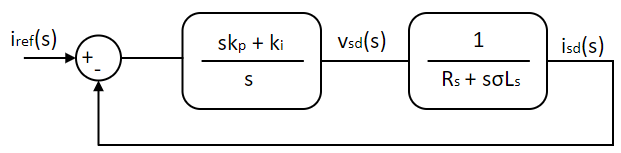The ki,p and ki,i gain values are adjusted to have the desired transient response. There are multiple ways to quantify the desired response. In time domain, usually the desired rise time and overshoot for a step input is mentioned and the gains are adjusted to satisfy this requirement. Alternatively, in frequency domain, the desired phase margin and cross-over frequency are mentioned. We shall go the latter route since it gives a better picture of the system stability. The steady state response of the system is obtained by substituting s = jω in Eqn. 9.

Kc(jω) = PIc(jω) × Gc(jω) = jωki,p + ki,i × 1jωσLs + Rs(27)

The above system is tuned for desired phase margin of Φi at cross-over frequency ωi,c as follows. At the cross-over frequency, the system gain equals 1 and the system phase shift equals -π + Φi. Substituting this into Eqn. 10:

∠Kc(jωi,c) = -π + Φi = -π2 + tan-1(ωi,cki,pki,i) - tan-1(ωi,cLsRs)

ωi,cki,pki,i = tan(Φi - π2 + tan-1(ωi,cLsRs))

⇒ ki,p = ki,iωi,c × tan(Φi - π2 + tan-1(ωi,cσLsRs)) = C1 × ki,i(28)

where,

C1 = 1ωi,c × tan(Φi - π2 + tan-1(ωi,cσLsRs))(29)

and

|Kc(jωi,c)| = 1 = |i,cki,p + ki,ii,c × 1i,cLs + Rs|

⇒ (ωi,cki,p)2 + k2i,i = ω2i,c((ωi,cLs)2 + R2a)

⇒ k2i,i= ω2i,c × i,cLs)2 + R2ai,c × C1)2 + 1(30)

Given the desired Φi and ωi,c, and the motor parameters, the PI gains can be solved using Eqns. 28 through 30.

Speed controller design

The mechanical system transfer function can be obtained from Eqn. 10. Ignoring the load torque as disturbance,

ωmech(s)Tem(s) = Gs(s) = 1sJ + B(31)

Using Eqn. 8, Eqn. 16 can be alternatively represented as given below, under vector control in steady state:

Tem = P2L2mLrisd,refisq = kt × isq(32)

The inner current loop can be modelled as unity gain transfer function. This assumption is valid as long as the response time of inner current loop is magnitude higher than the outer speed loop. Or in other words, the cross-over frequency of inner current loop ωi,c >> cross-over frequency of outer speed loop ωs,c. Similar to current controller, the above system can be represented as shown below:

Ks(jω) = PIs(jω) × Gs(jω) = ks,ps + ks,is × ktJs + B(33)

The above system is similar to that of the current controller designed earlier and going through the same procedure, the speed controller's gains are obtained to be:

ks,p = C2 × ks,i(34)

where,

C2 = 1ωs,c × tan(Φs - π2 + tan-1(ωs,cJB))(34)

and

k2s,i= (ωs,ckt)2 × s,cJ)2 + B2s,c × C2)2 + 1(35)

where, ks,p and ks,i are the speed Pi controller's proportional and integral gain.

##### Simulation of Induction motor speed control

The above speed control model is simulated using Workbench in this section.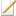Note

Save the model at frequent steps.

Open loop motor model

1. Create a new project, and add a new model and script file to the project.

2. Set this model file as the Start model/Function in project properties.

3. Open the script file and initialize all the model parameter as shown below from the parameters estimated in previous experiment.

Code
```Public Module IM
Public f As Native Double = 50 ! Rated frequency
Public Rs As Native Double = 1.79 ! Stator Resistance
Public Rr As Native Double = 1.05 ! Rotor Resistance
Public Lls As Native Double = 5E-3 ! Stator leakage Inductance
Public Llr As Native Double = 5E-3 ! Rotor leakage Inductance
Public Lm As Native Double = 30E-3 ! Mutual Inductance
Public Ls As Native Double = Lm + Lls ! Stator Inductance
Public Lr As Native Double = Lm + Llr ! Rotor Inductance
Public J As Native Double = 150E-6 ! Rotor Inertia
Public B As Native Double = 100E-6 ! Coefficient of Viscous friction
Public P As Native Double = 4 ! Number of poles
Public Vllrms As Native Double = 14.7 ! Rated line to line voltage
Public slip As Native Double = 0.1 ! Rated slip
Public ωsyn As Native Double = 2 * π * f ! Synchronous speed at rated frequency
Public ωm As Native Double = (1 - slip) * ωsyn ! Speed at rated slip
Public Va As Native Double = VLLrms * √2 / √3 ! Phase voltage peak
Public τr As Native Double = Lr / Rr
End Module
```
4. Open the model file and within its properties set the Step time to 5E-5.

5. Drag and drop a subsystem tool from the Toolbox which is present in Signal Routing tool class.

6. Subsystem is merely an enclosure within which more tools can be added. Double click the subsystem to open it.

7. Drag and drop the following tools from the Toolbox to the subsystem model and connect them as shown to create abc to dq transformation, given in Eqn. 12.

# Tool type Tool class Tool name
1 In-port Signal Routing I
2 In-port Signal Routing I1
3 Constant Signal Sources Constant
5 Elementary Function Math Block ElemF
6 Elementary Function Math Block ElemF1
7 Multiplier Divider Math Block Mul
8 Multiplier Divider Math Block Mul1
9 Demux Signal Routing DeMux
10 Demux Signal Routing DeMux1
13 Gain Math Block Gain
14 Gain Math Block Gain1
15 Concatenate Matrix Manipulation Concat
16 Out-port Signal Routing O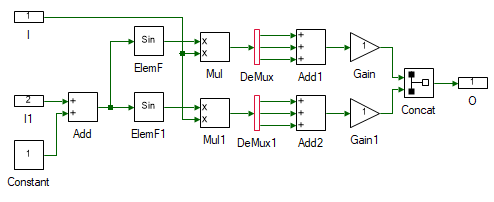8. Change the properties of each tool to values listed in table below.

Tool name Property field Property value Comments
I Name abc Input abc signal.
I1 Name theta Input theta.
Constant Magnitude {0, 2 * π / 3, 4 * π / 3} Muxed 3Φ phase shift signal.
ElemF Function Cos
ElemF1 Function Sin
DeMux Number of Ports 3 Split the muxed signal to independent signal.
DeMux1 Number of Ports 3 Split the muxed signal to independent signal.
Gain Gain √2 / √3 Scale by sqrt(2/3).
Gain1 Gain -(√2) / √3 Scale by -sqrt(2/3).
Concat Pivot Dimension 2 Combine the signal to form a column matrix.
O Name dq Output dq.

9. Go back to the main motor model by clicking on the tab on the top.

10. Resize the subsystem so that all the ports can be seen. A tool can be resized by clicking and dragging any of the four squares in the tool's corner.

11. Drag and drop the following tools from the Toolbox to the model and connect them as shown to test if abc to dq is functioning as expected.

# Tool type Tool class Tool name
1 Sine Signal Sources Sine
2 Constant Signal Sources Constant
3 Integrator Math Block Integrator
4 Mag-Time Scope Display Scope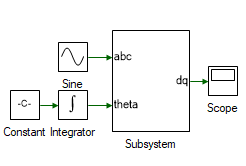12. Change the properties of each tool to values listed in table below.

Tool name Property field Property value Comments
Sine Phase shift (in degrees) {0, -120, -240} Input abc signal.
Constant Magnitude 2 * π * 1 Frequency of input signal in rads/s.

13. Run the simulation and check if the results are dc value, with d-axis equal to zero and q-axis negative.

14. Drag and drop the following tools from the Toolbox to the subsystem model and connect them as shown to create dq to abc transformation.

# Tool type Tool class Tool name
1 In-port Signal Routing I
2 In-port Signal Routing I1
3 Constant Signal Sources Constant
4 Demux Signal Routing DeMux
6 Elementary Function Math Block ElemF
7 Elementary Function Math Block ElemF1
8 Multiplier Divider Math Block Mul
9 Multiplier Divider Math Block Mul1
11 Gain Math Block Gain
12 Out-port Signal Routing O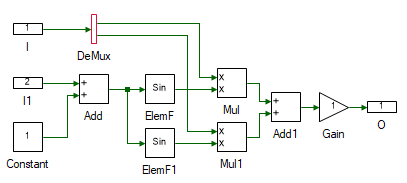15. Change the properties of each tool to values listed in table below.

Tool name Property field Property value Comments
I Name dq Input dq signal.
I1 Name theta Input theta.
Constant Magnitude {0, 2 * π / 3, 4 * π / 3} Muxed 3Φ phase shift signal.
ElemF Function Cos
ElemF1 Function Sin
Add1 Arithmetic operation +- Subtract the cosine and sine terms.
Gain Gain √2 / √3 Scale by sqrt(2/3).
O Name abc Output abc.

16. Go back to the main motor model by clicking on the tab on the top.

17. Resize the subsystem so that all the ports can be seen. A tool can be resized by clicking and dragging any of the four squares in the tool's corner.

18. Drag and drop the following tools from the Toolbox to the model and connect them as shown to test if abc to dq is functioning as expected. The output of ABCtoDQ is a column matrix and input of DQtoABC is muxed signal. Hence these must be converted.

# Tool type Tool class Tool name
1 Decatenate Matrix Manipulation Decat
2 Mux Signal Routing Mux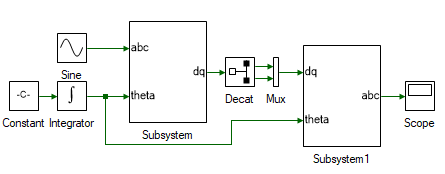19. Change the properties of each tool to values listed in table below.

Tool name Property field Property value Comments
Decat Pivot Dimension 2 Split along the row

20. Run the simulation and check if the results are same as the input.

21. Delete the Decat and Mux tool.

22. Next step is to determine the stator flux of the motor as given in Eqn. 13. Create another subsystem and drag and drop the tools shown and connect them as shown to implement the above equation.

# Tool type Tool class Tool name
1 In-port Signal Routing I
2 In-port Signal Routing I1
3 In-port Signal Routing I1
4 Constant Signal Sources Constant
5 Multiplier Divider Math Block Mul
6 Multiplier Divider Math Block Mul1
7 Gain Math Block Gain
9 Integrator Math Block Integrator
10 Out-port Signal Routing O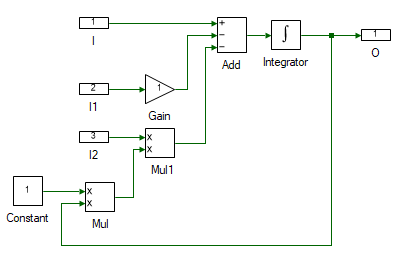23. Change the properties of each tool to values listed in table below.

Tool name Property field Property value Comments
I Name vsdq Stator voltage dq signal, vsdq.
I1 Name isdq Stator current dq signal, isdq.
I2 Name wd d-axis speed, ωd.
Gain Gain IM:Rs Stator resistance, Rr.
Constant Magnitude [[0, -1], [1, 0]] Rotational matrix
Integrator Initial value [, ] Initial stator flux linkage.
O Name fsdq Stator dq flux, λsdq.

24. Next step is to determine the stator and rotor currents from the flux. as given in Eqn. 6 through 10. Drag and drop the tools shown and connect them as shown to implement the above equation.

# Tool type Tool class Tool name
1 Constant Signal Sources Constant1
2 Concatenation Matrix Manipulation Concat
3 Concatenation Matrix Manipulation Concat1
4 Constant Signal Sources Constant2
5 Multiplier Divider Math Block Mul
6 Decatenation Matrix Manipulation Decat
7 Decatenation Matrix Manipulation Decat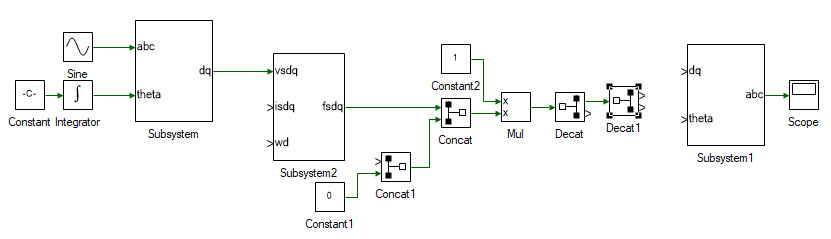25. Change the properties of each tool to values listed in table below.

Tool name Property field Property value Comments
Concat Pivot Dimension 2 Combine λsd and λsq.
Concat1 Pivot Dimension 2 Combine λrd and λrq.
Constant1 Magnitude 0 λrq = 0.
Constant2 Magnitude 1/[[IM:Ls, 0, IM:Lm, 0], [0, IM:Ls, 0, IM:Lm], [IM:Lm, 0, IM:Lr, 0], [0, IM:Lm, 0, IM:Lr]] Eqns. 6 through 10.
Decat Pivot Dimension 2 Split into isdq and irdq.
Decat Pivot Dimension 2 Split into isd and isq.

26. Next step is to determine the rotor flux and d-axis speed to close the model loop. Same as before add the following tools and connect them as shown.

# Tool type Tool class Tool name
1 Gain Math Block Gain
3 Integrator Math Block Integrator1
4 Gain Math Block Gain1
5 Multiplier Divider Math Block Mul1
6 Multiplier Divider Math Block Mul2
7 Gain Math Block Gain2
8 Gain Math Block Gain3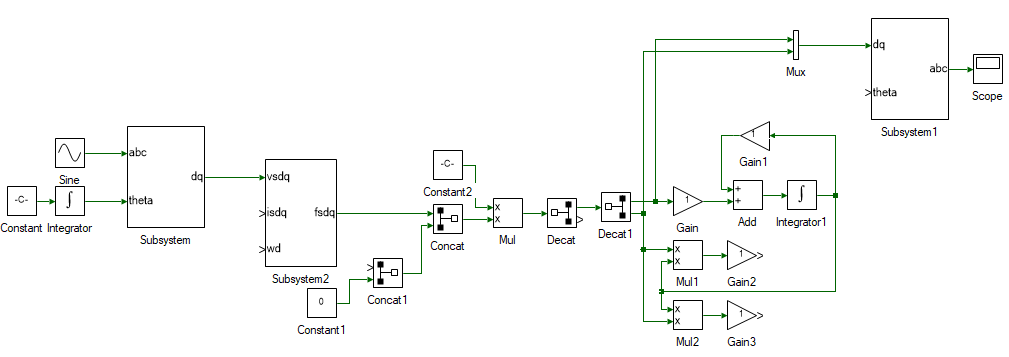27. Change the properties of each tool to values listed in table below.

Tool name Property field Property value Comments
Gain Gain IM:Lm / IM:τr Eqn. 14. λrd calculation.
Add Arithmetic operation -+ Eqn. 14. λrd calculation.
Gain1 Gain 1 / IM:τr Eqn. 14. λrd calculation.
Integrator1 Initial value 0.001 Eqn. 14. To prevent divide by zero error. Output of this is λrd.
Mul1 Arithmetic operation x/ Eqn. 15. ωdA calculation.
Gain2 Gain IM:Lm / IM:τr Eqn. 15. ωdA calculation. Output of this is ωdA.
Gain3 Gain (IM:p / 2) * IM:Lm / IM:Lr Eqn. 16. Torque caculation. Output of this is Tem.

28. The last remaining step is to determine the rotor speed using Eqn. 10. From the rotor speed and ωdA, the rotor flux axis speed is obtained using Eqn. 11. To do this add the following tools and connect them as shown.

# Tool type Tool class Tool name
1 Constant Signal Sources Constant3
3 Transfer function Continuous TransferFnc
4 Gain Math Block Gain4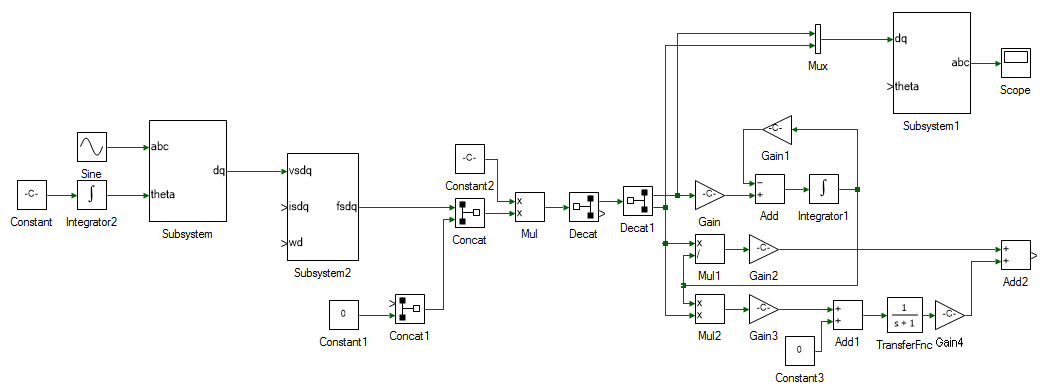29. Change the properties of each tool to values listed in table below.

Tool name Property field Property value Comments
Constant3 Magnitude 0 Eqn. 10. Load torque Tl.
Add Arithmetic operation +- Eqn. 10. Tl - Tem.
TransferFnc Numerator {1} Eqn. 10 can be represented in transfer function form as (Tem - TL)/(sJ + B).
TransferFnc Denominator {IM:J, IM:B} Eqn. 10 can be represented in transfer function form as (Tem - TL)/(sJ + B). Output of this block is the motor mechanical speed, ωmech.
Gain5 Gain IM:p / 2 ωm = P/2 x ωmech.
Add2 Arithmetic operation ++ ωd = ωm + ωdA.

30. All that remains is to close the loop. Delete the Constant tool connected to the abc → dq subsystem. This was initially used as the d-axis speed and is no longer needed since we have the actual d-axis speed, aligned with rotor flux. Connect the output of Add2 which is ωd to input of Integrator2. Output of Integrator2 is θda, which must be connected to dq → abc subsystem's theta input. Also connect the output of Add2, ωd to ωd input of stator flux subsystem. The stator dq currents for the stator flux subsystem, isdq is obtained by connecting the output of Decat. Finally, the rotor d-axis flux, λ`rd`, i.e. output of Integrator1 is connected to input of Concat1. The overall induction motor dq model, with d-axis aligned with rotor flux, is shown below.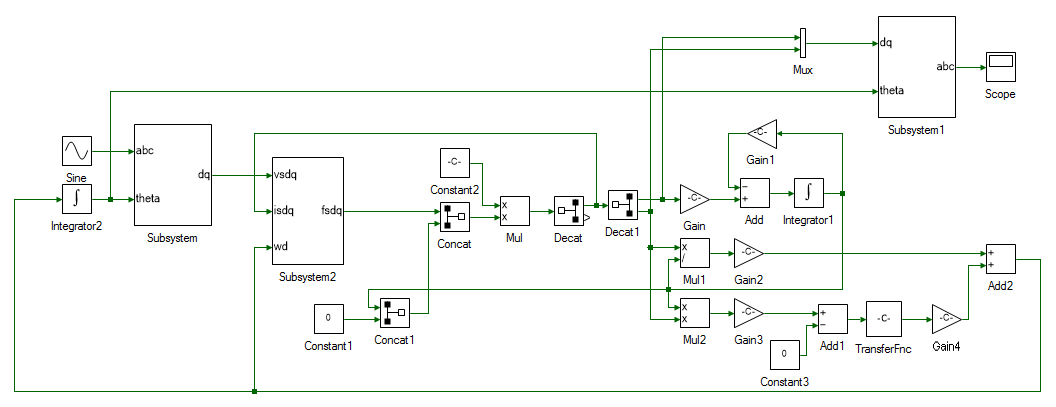31. Set the input voltage and frequency in the Sine block.

Tool name Property field Property value Comments
Sine Magnitude IM:Va Rated peak phase voltage
Sine Frequency (Hz) IM:f Rated frequency

32. Set the model Run time to 3.

33. Add scope to observe, abc and dq stator voltages and currents, motor speed, electromagnetic torque and any other values that might be of interest.

34. Run the simulation and observe the results.

35. Obtain results for the following operating conditions.

1. Input frequency = 0.5 x Rated frequency, Input voltage = 1 x Rated voltage

2. Input frequency = 1 x Rated frequency, Input voltage = 0.5 x Rated voltage

3. Input frequency = 0.5 x Rated frequency, Input voltage = 0.5 x Rated voltage

4. Input frequency = Rated frequency, Input voltage = Rated voltage

36. Replace the constant load torque, with a Step from Signal Sources. Set the Final value of step to 0.1 and Step time as 2.

37. Re-run the simulation and observe the results.

If any runtime exception occurs, use the DataPeek window, docked on the right, to look at each of the tool's input, output and parameter right before the exception occurred. This tool can be used to observe the value of any tool or code variables at any time. Click on the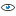icon in the DataPeek window and select any tool to look at its value. Similarly, to look at the value of a variable in Script file, place the cursor on the variable and select it by double clicking. Its value will pop-up in the DataPeek window.

Motor current control

In this section, the current through the motor is controlled using a PI controller.

1. Compute the ki,p and ki,i using Eqns. 28 through 30 for the system to have phase margin Φi = π/3 at cross-over frequency ωi,c = 2π x 200. The cross-over frequency is chosen as 200 Hz, which is < 1/10ᵗʰ the switching frequency of 6 kHz. This is merely a thumb rule, where every additional loop is 1/10ᵗʰ or less than the bandwidth of inner loop. In this case, it helps prevent the current controller from trying to compensate the switching current harmonics.

2. Open a new instance of Workbench.

3. In the motor model designed earlier, the motor currents and the speed are determined based on applied terminal voltage and load torque. These two parameters are measured using current and speed sensors for sensored-vector control. From these two parameters the rotor flux position is established using Eqn. 11 and 15 as already implemented earlier within the motor model. The control voltages are generated in dq frame and this must be converter to abc voltages to be generated by the power processing unit. This dq-abc transformation has been implemented earlier as well.

These two blocks are already implemented and can be opened from the examples folder usually in C:\Program Files (x86)\Sciamble\WorkBench v1\Examples\CUSPLab\AdvancedDrives\Experiment4\CurrentController. This motor model subsystem is the model that was built in the previous section and has been enclosed within a subsystem. In addition, the abc-dq transformation and the estimator subsystem have been added to the model file.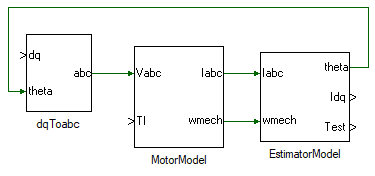4. Add another subsystem to the model, where the d-axis PI current controller will be modelled. Double click and open the subsystem.

5. Drag and drop the following tools from the Toolbox to the subsystem model and connect them as shown.

# Tool type Tool class Tool name
1 In-port Signal Routing I
2 In-port Signal Routing I1
4 Gain Math Block Gain
5 Gain Math Block Gain1
6 Integrator Math Block Integrator
8 Saturation Non-linear Saturation
9 Out-port Signal Routing O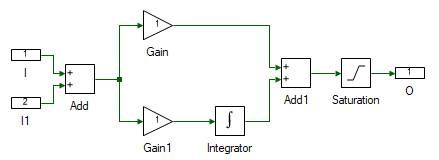6. Change the properties of each tool to values listed in table below.

Tool name Property field Property value Comments
I Name Ref Desired reference motor current.
I1 Name Fbk Actual motor current.
Add Arithmetic operation +- Difference between the desired and actual motor current.
Gain Gain ki,p Substitute the value of ki,p computed in step 1.
Gain1 Gain ki,i Substitute the value of ki,i computed in step 1.
Integrator Integrator Type Anti-windup Reason explained later.
Integrator Upper Limit 20
Integrator Lower Limit -20
Saturation Upper Limit 20
Saturation Lower Limit -20
O Name Out Applied terminal voltage to generate the desired current profile.

When there is a large difference between the desired current and the actual current, the PI controller produces a large output voltage to quickly correct this error. In real-world, the magnitude of this voltage is limited either due to available DC bus voltage or due to motor and power electronics voltage ratings. Hence in practical implementation of PI controller, the output of the PI controller is saturated to the maximum possible voltage. So, during transient conditions, when the actual current is different from the desired current, the Integrator in the PI controller rapidly builds up its output to a large value due to persistent input error. When the error does goes back to zero, this large output built up by the integrator does not die down and cause the actual current to overshoot the desired the value, causing the error to go negative, which causes the integrator output to fall, but it will end up falling by much larger value than desired causing the current to undershoot. This keeps repeating, and this oscillation will die down slowly. To avoid this, the value up to which integrator is active is clamped by setting it to Anti-windup. If the integrator output reaches this value, the integrator is disabled until the polarity of the error reverses. This improves the response of the system significantly.

7. Create a copy of the PI subsystem for q-axis current control. Connect the output of these two PI controllers to the dq to abc subsystem as shown: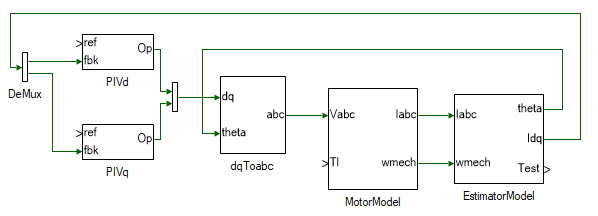8. Set the d-axis current reference to a constant obtained by solving Eqns. 17 through 21.

9. Set the q-axis current reference to step from 0 A to 1 A at time t = 2s.

10. Add a step load torque and for the time being set the Final value to 0.

11. Run the simulation and observe if the steady state dq currents match the reference currents as well if the transient response is satisfactory.

Motor speed control

In this section, the speed of the motor is controlled using a PI controller.

1. Compute the ks,p and ks,i using Eqn. 14 through 17 for the system to have phase margin Φs = π/3 at cross-over frequency ωs,c = ωi,i/10 = 2π x 20. The cross-over frequency is chosen as 20 Hz, which is 1/10ᵗʰ the cross-over frequency of inner current loop.

2. Replace the step source for q-axis current reference with a PI controller subsystem, output of which will be the reference q-axis current.

3. Change the PI gains to the values computed in the earlier step. Saturate the integrator and the PI subsystem's output to 5, which is the transient current limit of the motor under test.

4. Connect the feedback input to motor speed from the motor model subsystem. Apply a step speed for reference, stepping from 0 to 100 rads/s at time t = 2s.

5. Change the Final value of load torque to 0.05 Nm at time t = 4 s.

6. Run the simulation and observer if motor steady state speed matches the reference speed.

This concludes the simulation of induction motor vector control. In the following section, a pre-built induction motor vector control model is run in real-time.

##### Real-time vector control of Induction motor
1. Open Workbench and pin the Explorer and Properties dock.

2. Navigate to and open the IMVectorControl project file in Experiment4\Realtime folder usually in the following location: C:\Program Files (x86)\Sciamble\WorkBench v1\Examples\CUSPLab\AdvancedDrives.

3. Expand the project in the Explorer and open the ModelFile model file shown below: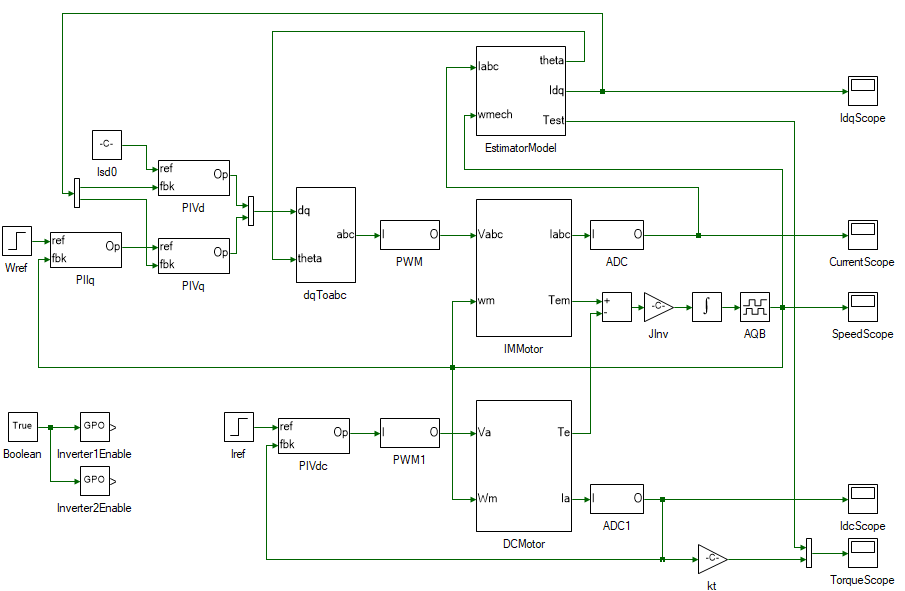The top section of the model consists of the induction motor vector control and is merely a replication of the simulation model developed earlier with few additions to enable real-time control. On the left is the speed PI controller, followed by the d and q axis current PI controller, the output of which is the dq terminal voltages. The dq voltages are converted to abc voltages in the following subsystem. In the simulation model, this terminal voltage signal was connected to the motor model. In this case it is converted to inverter's PWM duty cycles, to control the PMAC motor in real-time. In real-time mode, the stator currents are measured from the actual motor currents by means of ADCs and these are on the top-right corner of the model. The speed is measured from the actual motor's A-quad-B encoder. In simulation the rotor position is obtained by integrating the rotor speed. In real-time this is obtained from AQB position toolbox. The speed itself in real-time is obtained by actually differentiating the AQB position value. Hence integrating speed to obtain position will lead to loss of precision compared to just directly reading the position.

The bottom section consists of the DC motor current control. This is used to emulate the load torque.

4. The Init() function within the IMParam script file computes the controller parameters based on the motor parameters, and the loop cross-over frequency and phase margin. Set the speed loop cross-over frequency ωc and the phase margin φm in the script to the same value used in simulation.

5. The Init() function must be called prior to running the simulation model to initialize the controller gain values. To do this open project properties, double click on the IMVectorControl project node in the Explorer, and set the Prerun Model/Function to IMParam:Init() as shown below:

Similarly set the Start model/Function to ModelFile, to run the model after parameter initialization.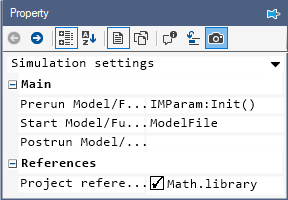6. Click the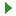button to run the model in simulation mode. Using the DataPeek, verify that the controller gain parameters computed by the Init() function matches that of values computed earlier.

7. Turn ON the DC power supply and set the voltage to 40 V.

8. Ensure that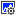button on the top dock, to transition to real-time mode, is pressed. Click the run button.Note

When the real-time mode button is pressed, the motor model gets grayed out. Tools that are grayed out indicate that they are not coded in real-time. Workbench can automatically identify tools that need to be coded in real-time and those that dont. This allows for using a single unified model for both real-time as well as simulation which generating a highly optimized real-time code.

9. Observe the speed and current result in the scope. Click the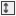to focus the result. After about 5 s, stop data logging by clicking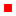.

10. Turn OFF the DC power supply.

11. If necessary, repeat the experiment for different loop cross-over frequencies and phase margins.

12. Turn OFF the DC power supply and disconnect all the connections including the USB.

##### Reference
1. "Advanced Electric Drives: Analysis, Control, and Modeling Using MATLAB/Simulink," Ned Mohan, Wiley Publication.

##### Lab report and reading assignment
1. List the kp and ki values of the current and speed PI controller.

2. Attach the plot of 3φ stator and rotor currents from simulation.

3. Attach the plot of the electromagnetic torque response, stator dq current, and the rotor speed from both simulation and real-time.

4. Ignoring the noise in the waveform in real-time mode, do the stator dq currents and the electromagnetic torque waveforms match those obtained from simulation? If not, explain why.

5. Read through and add comments to each line of code in the Init() function. Later experiments would require writing the script file to compute the controller parameters.